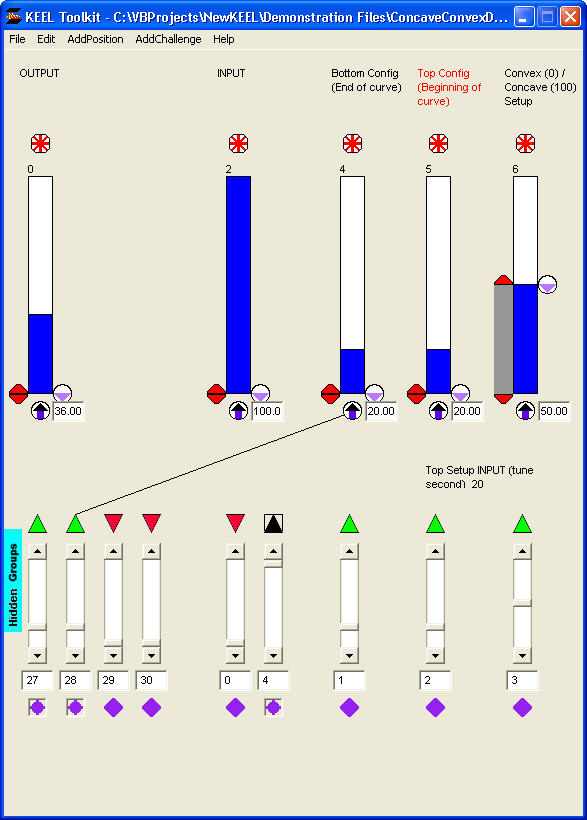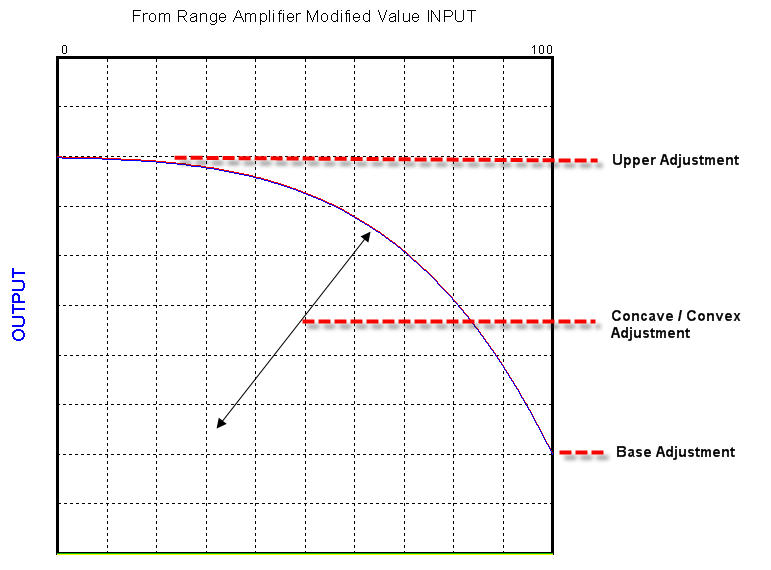Concave / Convex Decreasing

ConcaveConvexDecreasing.xml

Design

This technique shows the code segment for a curve that can exhibit concave or convex curves that decrease from left to right throughout the 0 to 100 input range. This is one of several complex curves that allow different shapes to be created by using input variables that can tune the shape of the curve.

With this curve the Bottom Config (End of curve) (index 1) and the Top Config (Beginning of curve) (index 2) can be set with input variables. When configuring these values to precise values using the Auto Tuning function, set the Bottom Config (End of curve) (index 1) first with the INPUT set to 100, then set the Top Config (Beginning of curve) (index 2) with the INPUT set to 0. The curve can also be changed from convex to concave using another input (index 3). When input (index 3) is set to 50, a straight line results.